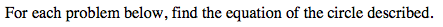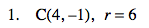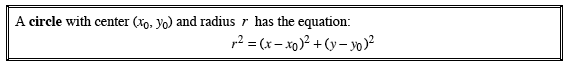### Home > PC > Chapter 13 > Lesson 13.1.1 > Problem13C-1

13C-1.x0 = 4y0 = −1

$6^2=(\textit{x}-4)^2+(\textit{y}-(-1))^2$